# Efficient Ray Tracing of Volume Data

Marc Levoy

First published in: ACM Transactions on Graphics, 9(3):245-261, July 1990Figure 1. Overview of volume rendering algorithm.Figure 2. Coordinate system used during volume rendering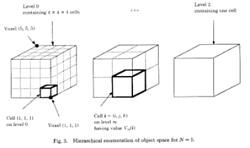Figure 3. Hierarchical enumeration of object space for N=5.Figure 4. Ray tracing of hierarchical enumeration.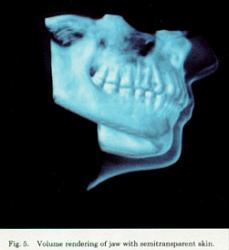Figure 5. Volume rendering of jaw with semitransparent skin.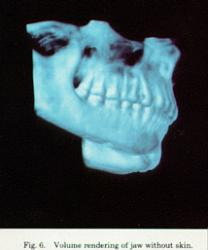Figure 6. Volume rendering of jaw without skin.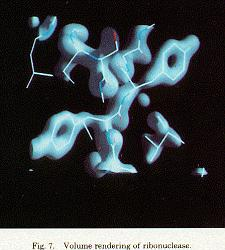Figure 7. Volume rendering of ribonuclease.Figure 8. Volume rendering of head.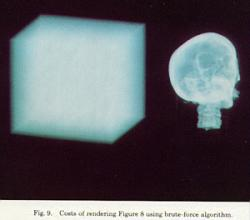Figure 9. Costs of rendering Figure 8 using brute-force algorithm.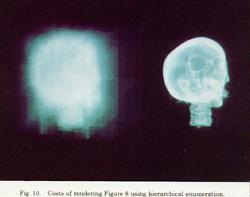Figure 10. Costs of rendering Figure 8 using hierarchical enumeration.Figure 11. Costs of rendering Figure 8 using hierarchical enumeration and adaptive termination.